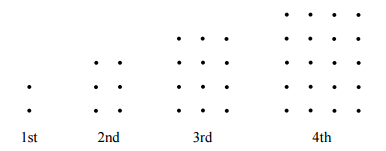# Another Rectangular Grid Problem

I drew rectangular grids with one more row than their column. The number of dots in the first four grids are 2, 6, 12, and 20, as shown in the diagram below:What is the total number of dots used in the first eleven grids?

×